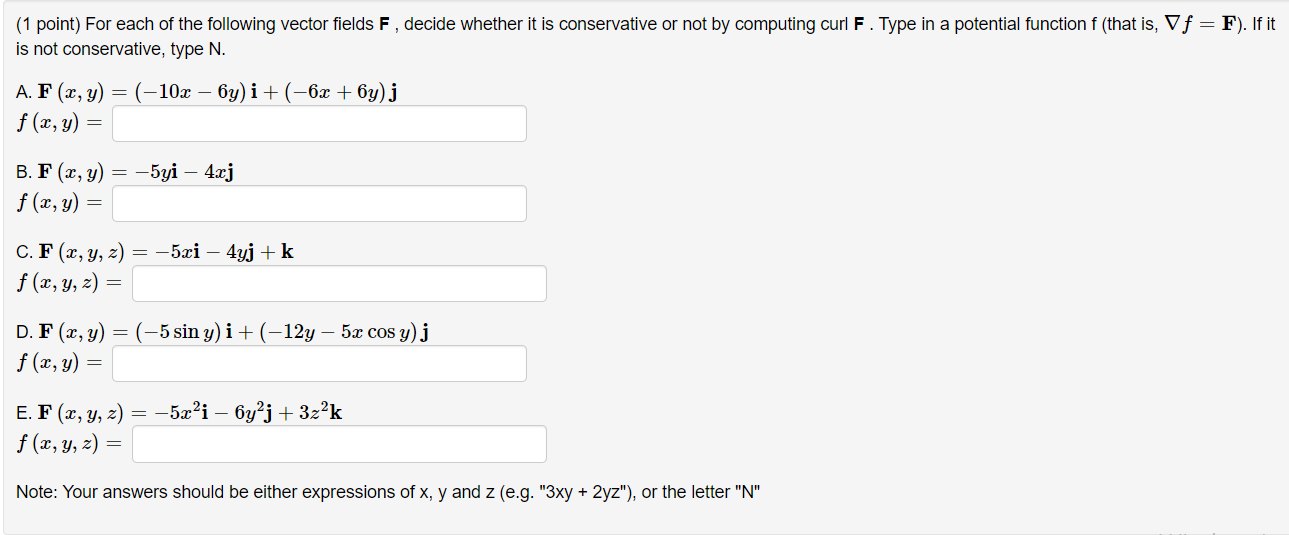### Create an Account

Already have account?

### Forgot Your Password ?

Home / Questions / (1 point) For each of the following vector fields F, decide whether it is conservative or ...

# (1 point) For each of the following vector fields F, decide whether it is conservative or not by computing curl F. Type in a potential function f (that is, V f = F). If it is not conservative, type N.

(1 point) For each of the following vector fields F, decide whether it is conservative or not by computing curl F. Type in a potential function f (that is, V f = F). If it is not conservative, type N. A. F(x, y) = (-10x – 6y) i +(-6x + 6y)j f (x,y) = B. F(x, y) = -5yi – 4xj f(x, y) = C. F(x, y, z) = -5xi – 4yj +k f (x, y, z) = D. F(x, y) = (-5 sin y) i + (-12y – 5x cos y) j f(x, y) = E. F(x, y, z) = -5x’i – 6y?j + 3z²k f (x, y, z) = Note: Your answers should be either expressions of x, y and z (e.g. "3xy + 2yz"), or the letter "N"Apr 14 2021 View more View Less

#### Answer (Solved)Subscribe To Get Solution